# NCERT Exemplar Solutions for Class 6 Maths Chapter 9 Symmetry and Practical Geometry

NCERT Exemplar Solutions for Class 6 Maths Chapter 9 Symmetry and Practical Geometry are available in PDF format. The complex problems assist students in developing problem-solving and higher-order thinking skills. It aims to provide students with a variety of quality problems in different formats, namely, multiple-choice questions, unique topics, skill-developing questions. Students who wish to score good marks in Maths practise NCERT Exemplar Solutions for Class 6 Maths.

In Chapter 9 – Symmetry and Practical Geometry, a figure is said to have line symmetry, if by folding the figure along a line, the left and right parts of it coincide exactly. The line is called the line (or axis) of symmetry of the figure. In this Chapter, students are going to learn about line symmetry, mirror reflection. Many constructions can be made using different instruments of a geometry box.

## Download the PDF of NCERT Exemplar Solutions for Class 6 Maths Chapter 9 Symmetry and Practical Geometry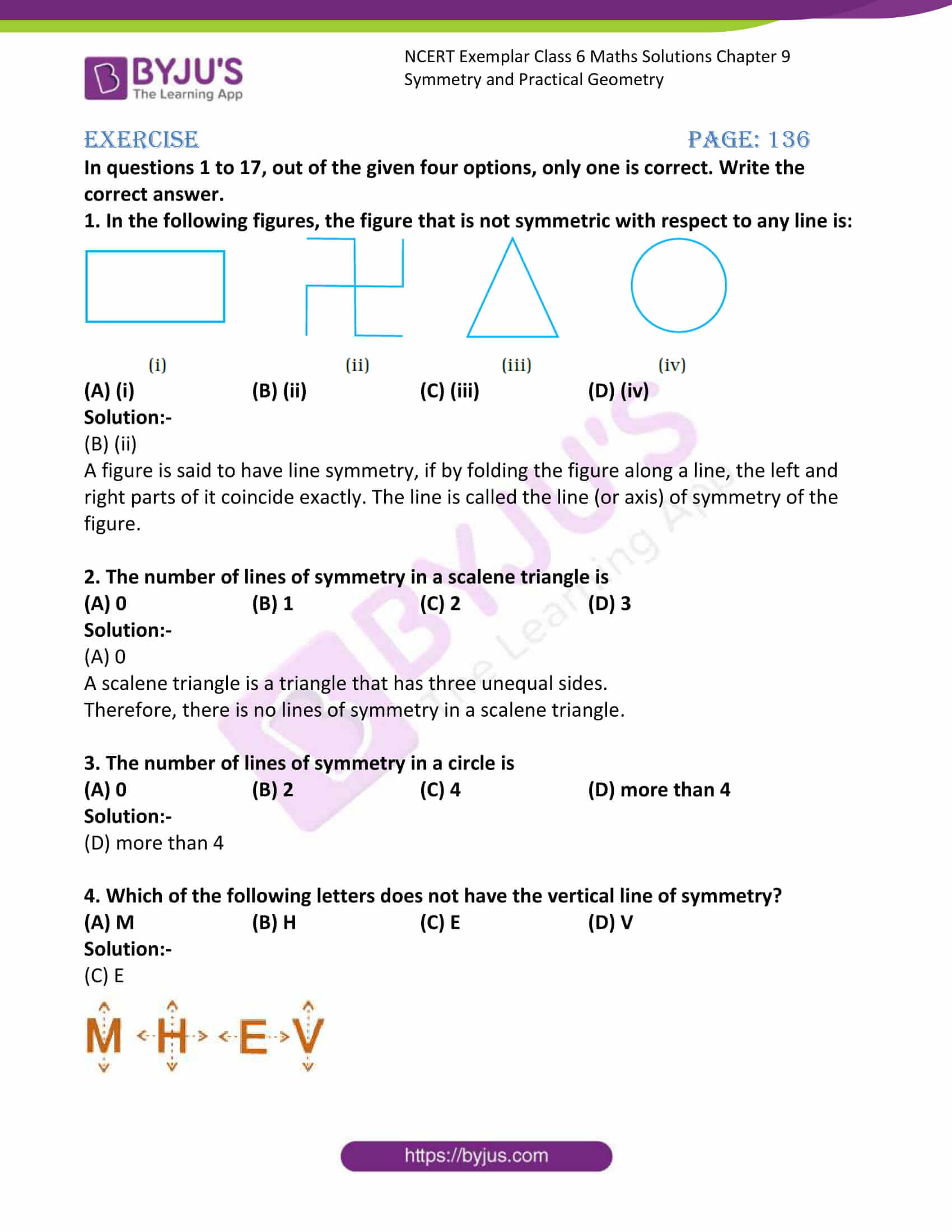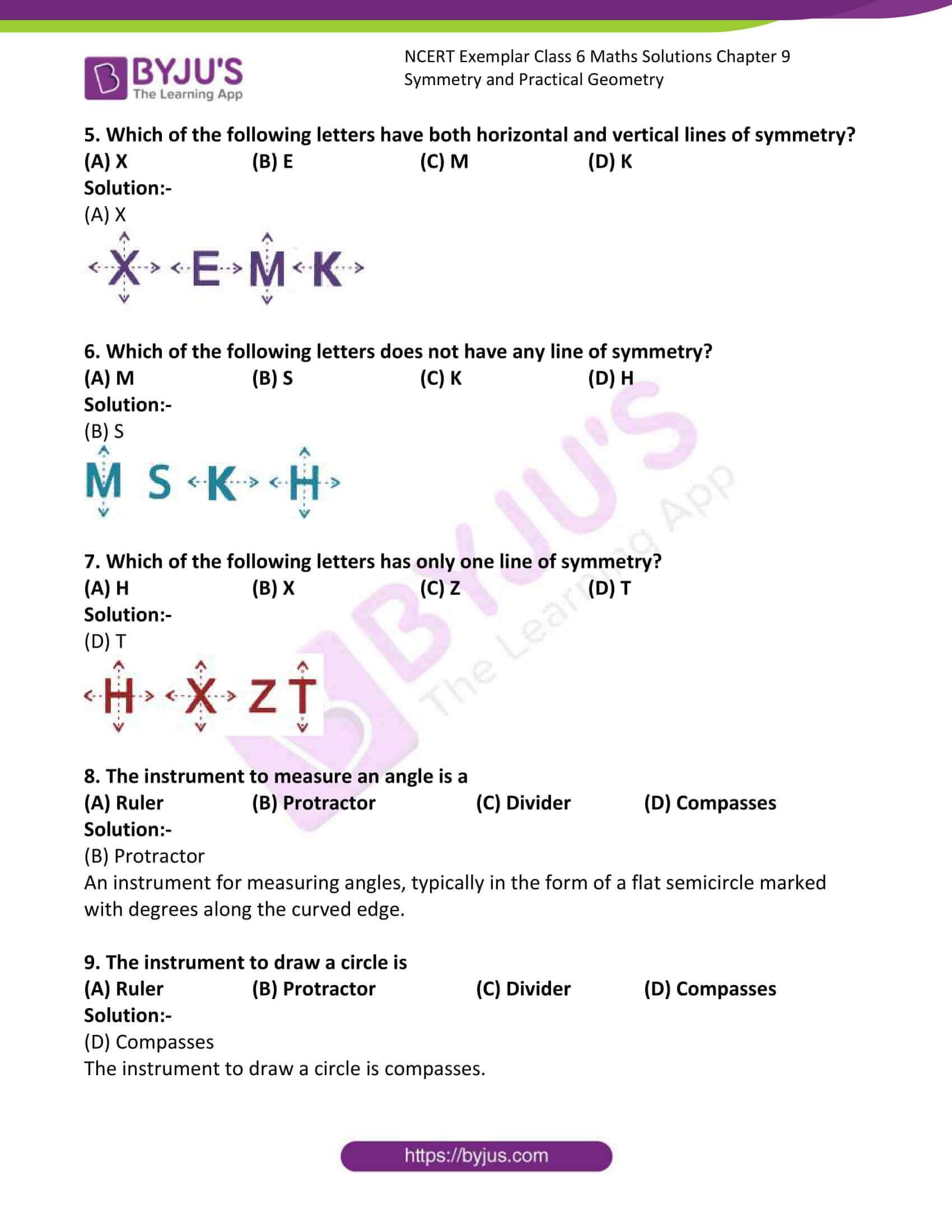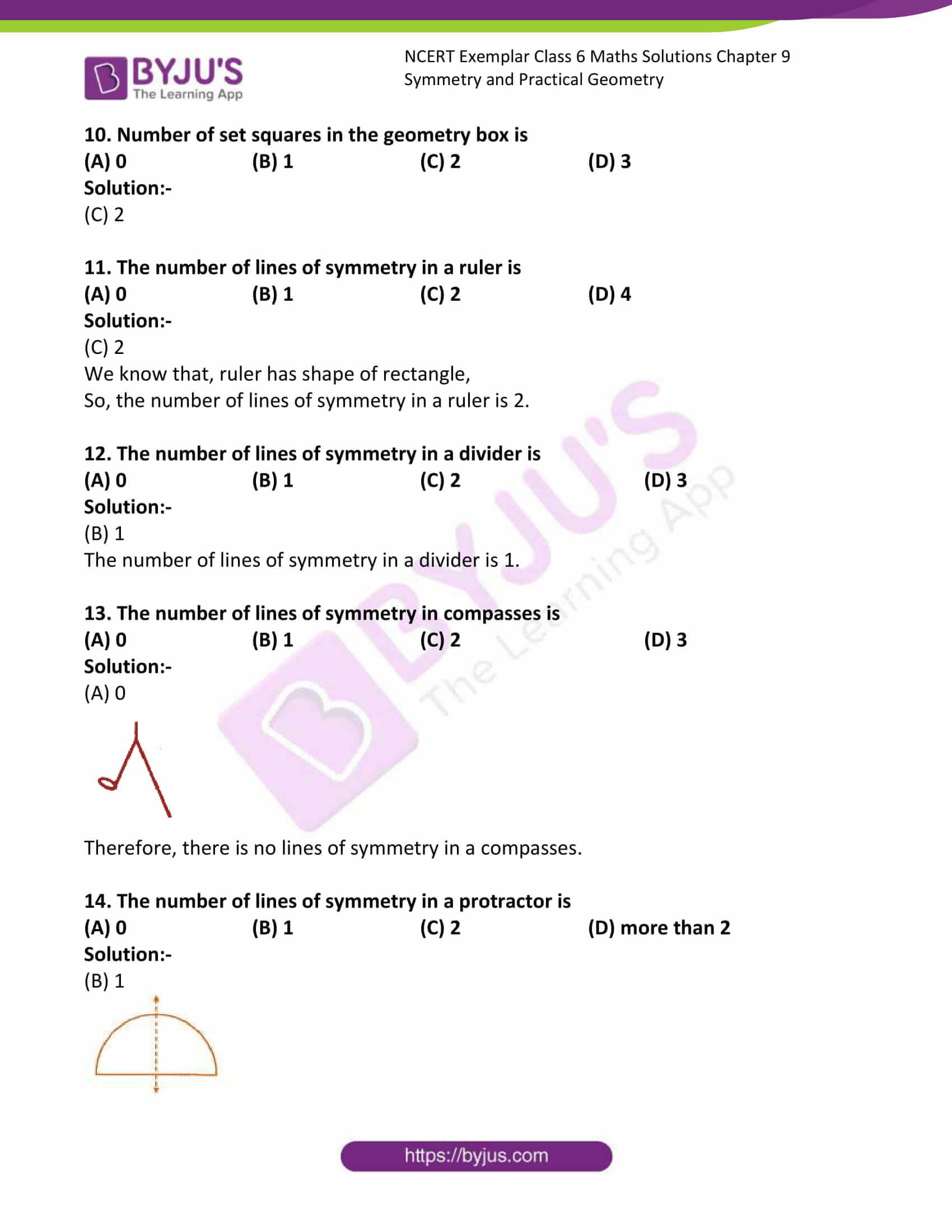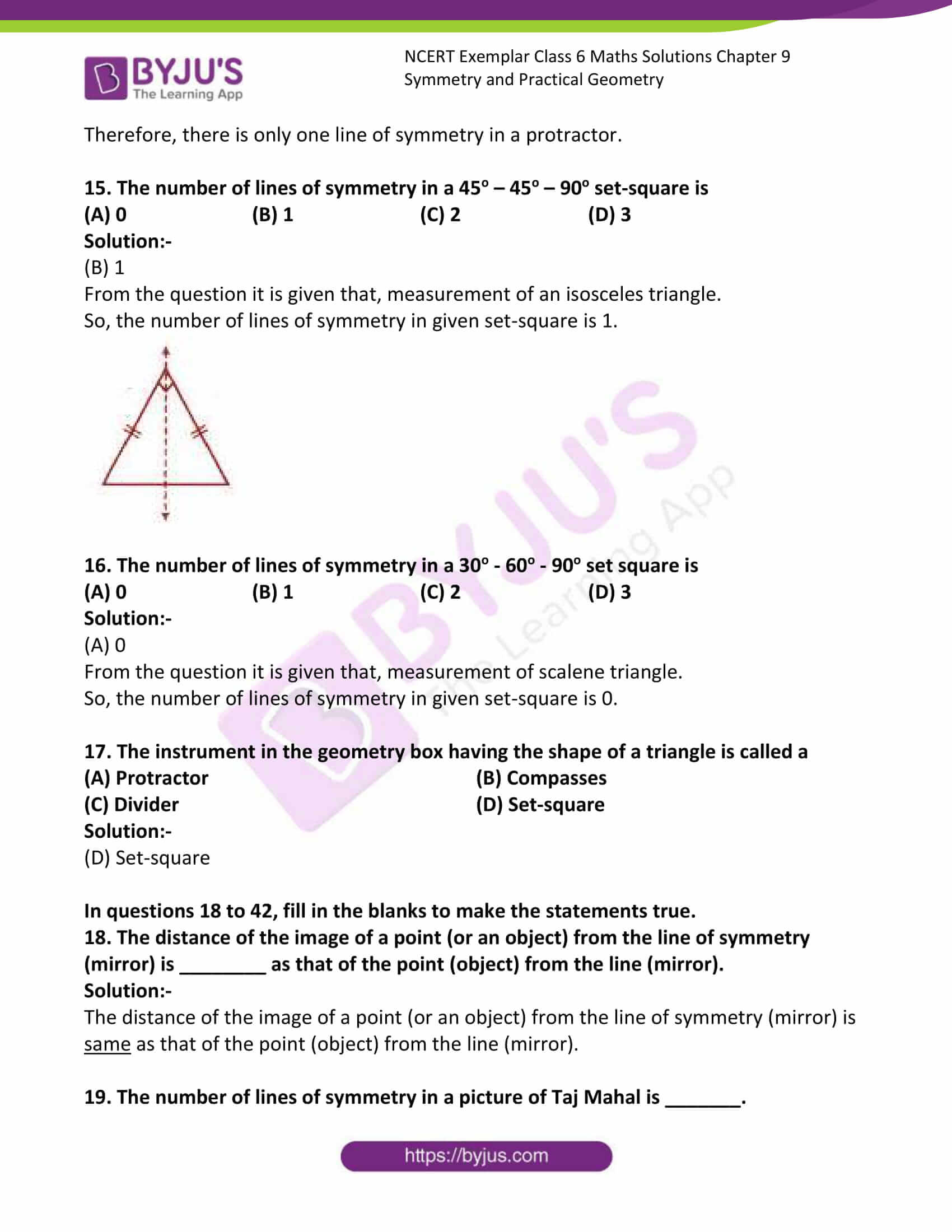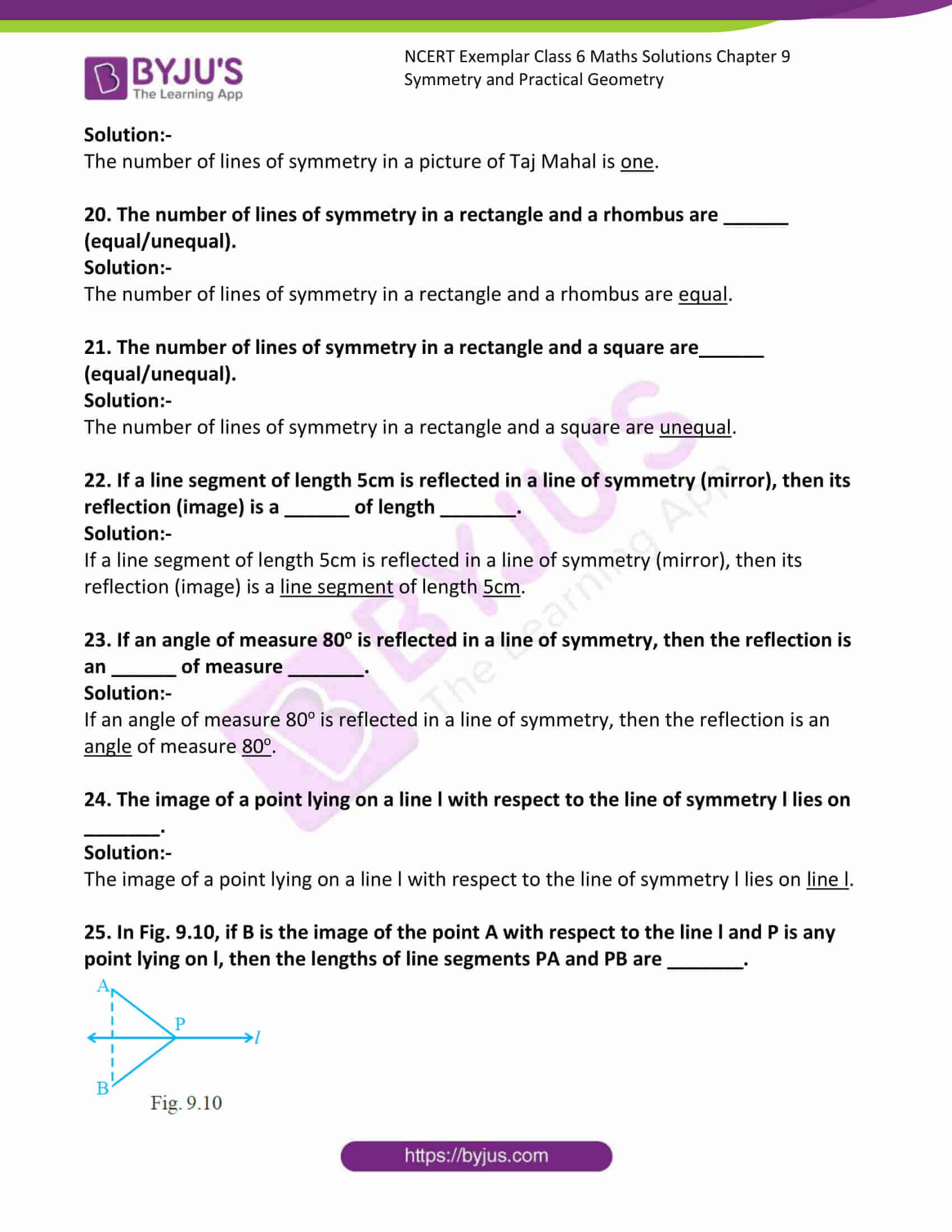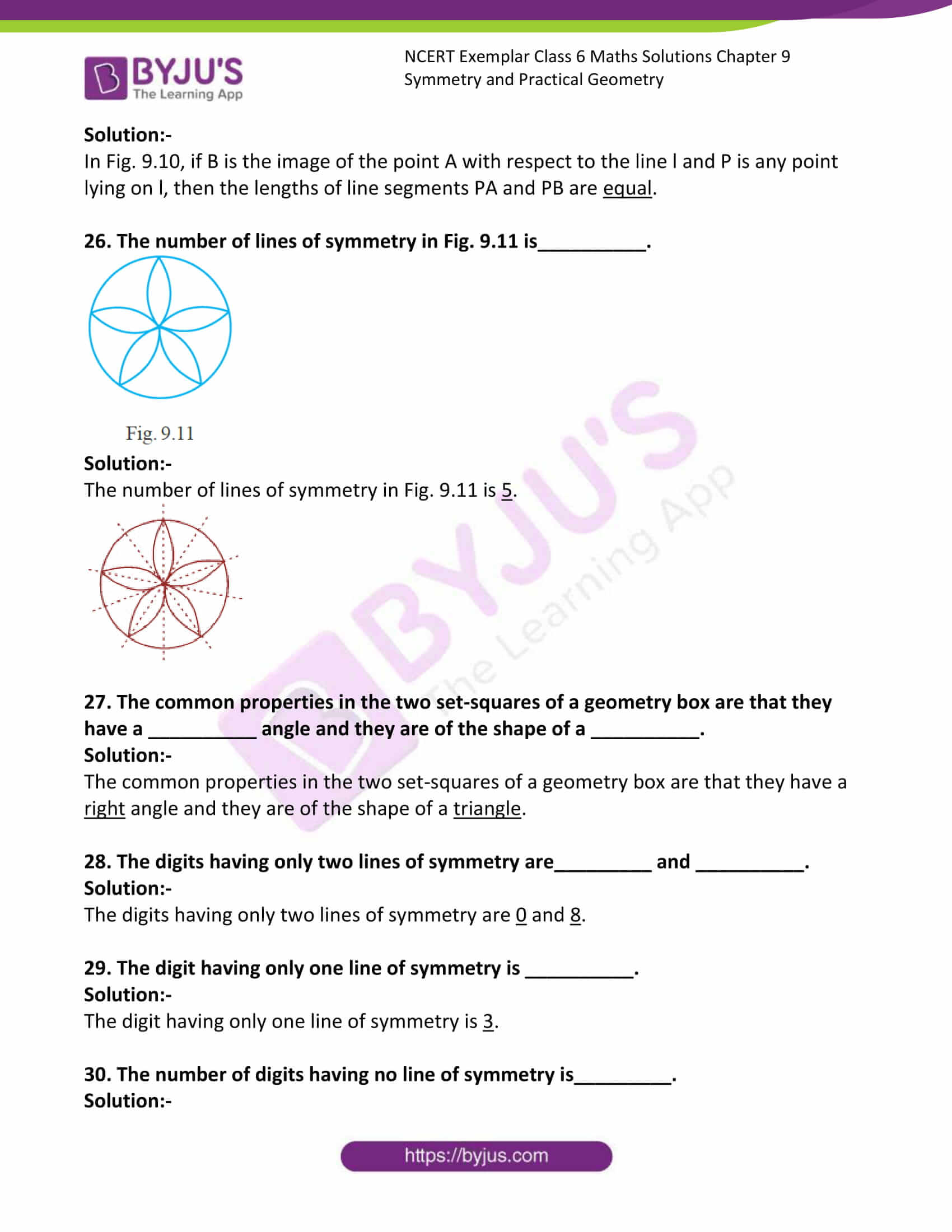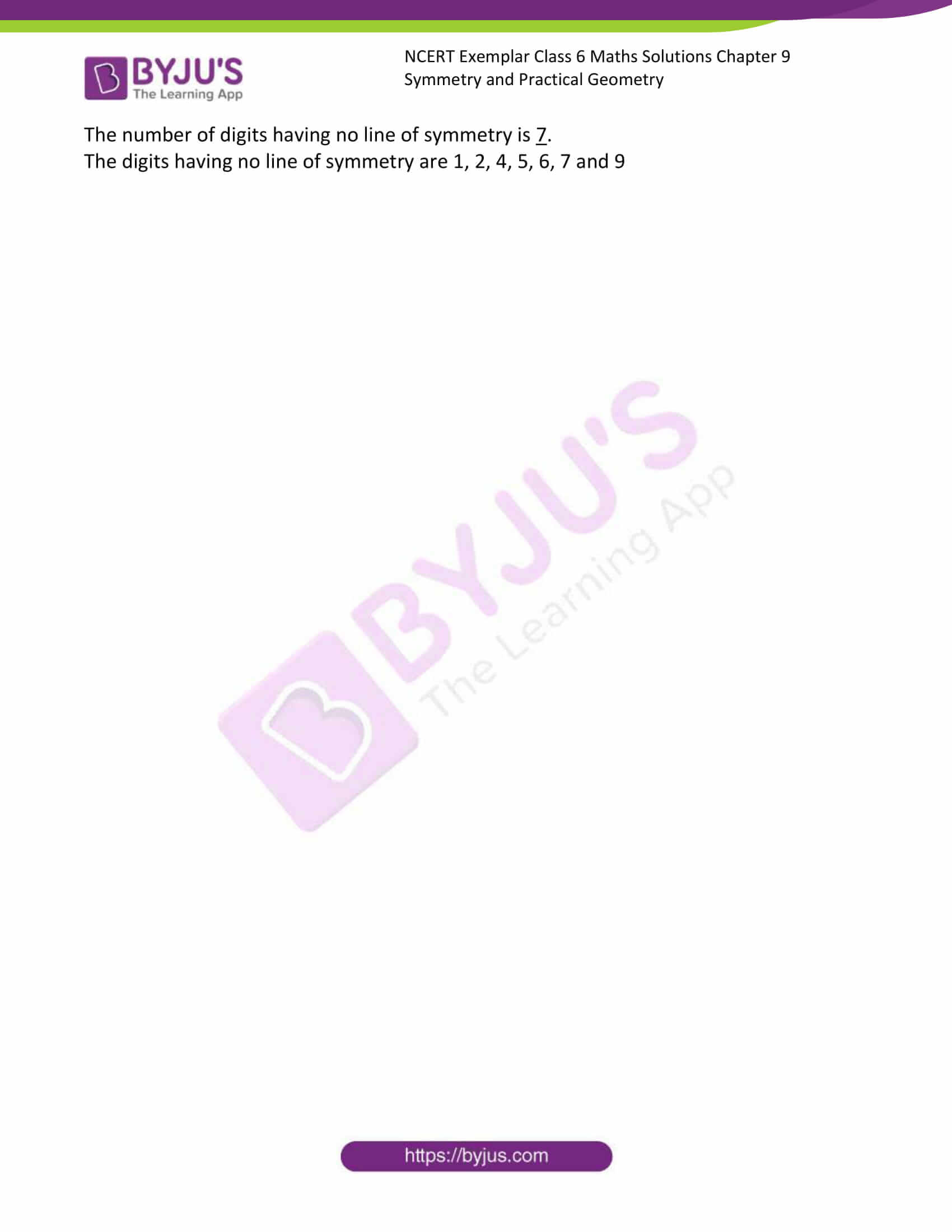### Access Answers to Maths NCERT Exemplar Solutions for Class 6 Chapter 9 Symmetry and Practical Geometry

Exercise Page: 136

In questions 1 to 17, out of the given four options, only one is correct. Write the correct answer.

1. In the following figures, the figure that is not symmetric with respect to any line is: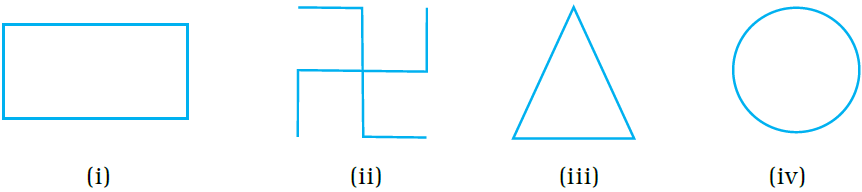(A) (i) (B) (ii) (C) (iii) (D) (iv)

Solution:-

(B) (ii)

A figure is said to have line symmetry, if by folding the figure along a line, the left and right parts of it coincide exactly. The line is called the line (or axis) of symmetry of the figure.

2. The number of lines of symmetry in a scalene triangle is

(A) 0 (B) 1 (C) 2 (D) 3

Solution:-

(A) 0

A scalene triangle is a triangle that has three unequal sides.

Therefore, there is no lines of symmetry in a scalene triangle.

3. The number of lines of symmetry in a circle is

(A) 0 (B) 2 (C) 4 (D) more than 4

Solution:-

(D) more than 4

4. Which of the following letters does not have the vertical line of symmetry?

(A) M (B) H (C) E (D) V

Solution:-

(C) E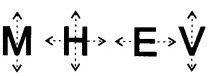5. Which of the following letters have both horizontal and vertical lines of symmetry?

(A) X (B) E (C) M (D) K

Solution:-

(A) X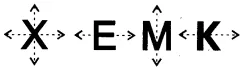6. Which of the following letters does not have any line of symmetry?

(A) M (B) S (C) K (D) H

Solution:-

(B) S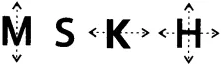7. Which of the following letters has only one line of symmetry?

(A) H (B) X (C) Z (D) T

Solution:-

(D) T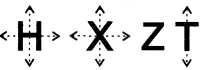8. The instrument to measure an angle is a

(A) Ruler (B) Protractor (C) Divider (D) Compasses

Solution:-

(B) Protractor

An instrument for measuring angles, typically in the form of a flat semicircle marked with degrees along the curved edge.

9. The instrument to draw a circle is

(A) Ruler (B) Protractor (C) Divider (D) Compasses

Solution:-

(D) Compasses

The instrument to draw a circle is compasses.

10. Number of set squares in the geometry box is

(A) 0 (B) 1 (C) 2 (D) 3

Solution:-

(C) 2

11. The number of lines of symmetry in a ruler is

(A) 0 (B) 1 (C) 2 (D) 4

Solution:-

(C) 2

We know that, ruler has shape of rectangle,

So, the number of lines of symmetry in a ruler is 2.

12. The number of lines of symmetry in a divider is

(A) 0 (B) 1 (C) 2 (D) 3

Solution:-

(B) 1

The number of lines of symmetry in a divider is 1.

13. The number of lines of symmetry in compasses is

(A) 0 (B) 1 (C) 2 (D) 3

Solution:-

(A) 0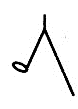Therefore, there is no lines of symmetry in a compasses.

14. The number of lines of symmetry in a protractor is

(A) 0 (B) 1 (C) 2 (D) more than 2

Solution:-

(B) 1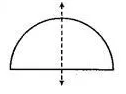Therefore, there is only one line of symmetry in a protractor.

15. The number of lines of symmetry in a 45o – 45o – 90o set-square is

(A) 0 (B) 1 (C) 2 (D) 3

Solution:-

(B) 1

From the question it is given that, measurement of an isosceles triangle.

So, the number of lines of symmetry in given set-square is 1.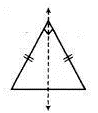16. The number of lines of symmetry in a 30o – 60o – 90o set square is

(A) 0 (B) 1 (C) 2 (D) 3

Solution:-

(A) 0

From the question it is given that, measurement of scalene triangle.

So, the number of lines of symmetry in given set-square is 0.

17. The instrument in the geometry box having the shape of a triangle is called a

(A) Protractor (B) Compasses

(C) Divider (D) Set-square

Solution:-

(D) Set-square

In questions 18 to 42, fill in the blanks to make the statements true.

18. The distance of the image of a point (or an object) from the line of symmetry (mirror) is ________ as that of the point (object) from the line (mirror).

Solution:-

The distance of the image of a point (or an object) from the line of symmetry (mirror) is same as that of the point (object) from the line (mirror).

19. The number of lines of symmetry in a picture of Taj Mahal is _______.

Solution:-

The number of lines of symmetry in a picture of Taj Mahal is one.

20. The number of lines of symmetry in a rectangle and a rhombus are ______ (equal/unequal).

Solution:-

The number of lines of symmetry in a rectangle and a rhombus are equal.

21. The number of lines of symmetry in a rectangle and a square are______ (equal/unequal).

Solution:-

The number of lines of symmetry in a rectangle and a square are unequal.

22. If a line segment of length 5cm is reflected in a line of symmetry (mirror), then its reflection (image) is a ______ of length _______.

Solution:-

If a line segment of length 5cm is reflected in a line of symmetry (mirror), then its reflection (image) is a line segment of length 5cm.

23. If an angle of measure 80o is reflected in a line of symmetry, then the reflection is an ______ of measure _______.

Solution:-

If an angle of measure 80o is reflected in a line of symmetry, then the reflection is an angle of measure 80o.

24. The image of a point lying on a line l with respect to the line of symmetry l lies on _______.

Solution:-

The image of a point lying on a line l with respect to the line of symmetry l lies on line l.

25. In Fig. 9.10, if B is the image of the point A with respect to the line l and P is any point lying on l, then the lengths of line segments PA and PB are _______.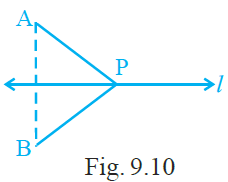Solution:-

In Fig. 9.10, if B is the image of the point A with respect to the line l and P is any point lying on l, then the lengths of line segments PA and PB are equal.

26. The number of lines of symmetry in Fig. 9.11 is__________.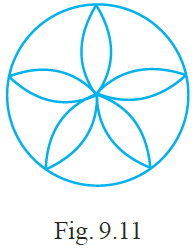Solution:-

The number of lines of symmetry in Fig. 9.11 is 5.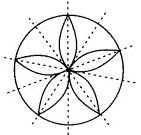27. The common properties in the two set-squares of a geometry box are that they have a __________ angle and they are of the shape of a __________.

Solution:-

The common properties in the two set-squares of a geometry box are that they have a right angle and they are of the shape of a triangle.

28. The digits having only two lines of symmetry are_________ and __________.

Solution:-

The digits having only two lines of symmetry are 0 and 8.

29. The digit having only one line of symmetry is __________.

Solution:-

The digit having only one line of symmetry is 3.

30. The number of digits having no line of symmetry is_________.

Solution:-

The number of digits having no line of symmetry is 7.

The digits having no line of symmetry are 1, 2, 4, 5, 6, 7 and 9